## Tuesday, April 10, 2012

### An Introduction to Social Network Analysis with R and NetDraw

With the rise in the use of social media, data related to social networks is ripe for analysis using techniques from social network analysis and graph theory. According to International Network for Social Network Analysis, ‘Social network analysis is focused on uncovering the patterning of people's interaction’.

Social network analysis (SNA) allows us to answer questions such as who are key  actors in a network? Who are the most influential members of a network? Who seems to be acting on the peripheral? Which connections in the network are most important?  Are there key players bridging connections or information between otherwise disconnected groups? Have policies or other forces changed the overall dynamics/interaction between people in the network (i.e. has the network structure changed in any meaningful way) and does that relate to some other performance outcome or goal?

More specific applications of SNA may include Student Integration and Persistence, Business to Business Supply Chains, and analysis of networks of terrorist cells.

Terminology and Mathematics of SNA

A network can be thought of in terms of graph theory as a set of vertices connected by ties. The vertices can include individuals, teams, government agencies, organizations, facebook group members, topics, patents,etc.  (Coulon,2005).  The ties, represented as lines connecting the vertices are referred to as ‘edges.’  Edges therefore indicate the connections between individuals, organizations etc.  Vertices and connections can be represented by what’s referred to as an adjacency matrix ‘A’, where Aij = 1 if there is an edge between vertices ‘i’ and ‘j’, and Aij = 0 otherwise.  Adjacency matrices are symmetric, in that Aij= Aji.

For example, the following adjacency matrix corresponds to the network depicted below: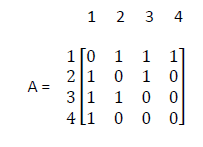Network: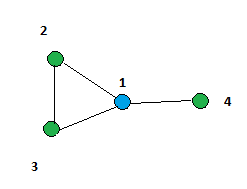Who’s Who in a Network?

Of interest is to use this mathematical representation of a network to quantify information that can be used to identify what we might call ‘key players’ in a network.  These measures include ‘betweenness’, ‘degree centrality’ and ‘eigenvector centrality.’

Betweenness is the number of shortest paths an actor is on (Conway, 2009).  For example, in the network depicted above, vertex 1 is on a path between 2 and 4 and another path between 3 and 4. Betweenness for vertex 1 is then equal to 2. There is another path between 2 and 4 via vertex 3, but it is not the shortest path, so it does not count. Similarly, 2 is on a path between 1 and 3, but its not as short as the direct path between 1 and 3.  Therefore, betweenness for the other vertices is zero, because all other vertices are at the end of a path, and are not on any of the shortest paths between vertices.

Degree Centrality is simply the number of connections (or edges) a vertex has to other vertices. Its clear from counting that #1’s degree centrality is 3, while vertex #4 has a measure of degree centrality equal to 1.

Eigenvector Centrality is a measure that reflects the fact that  not all connections are equal, and in fact, connections to people that are more influential are more important (Newman, 2012).  Mathematically, the measure of eigenvector centrality is derived from the weights that consist of the values from the leading eigenvector  (the eigenvector associated with the largest eigenvalue) of the adjacency matrix depicting the network.

Recall, the value λ is an eigenvalue (or vector of eigenvalues) of the matrix A, and x is an eigenvector of A if the following equation holds:

λ x = A x

So, for the network depicted above, the centrality measures for each vertex = 1,2,3,4 can be derived from the components (x1,x2,x3,x4) that comprise the eigenvector x.
Eigenvectors and eigenvalues can be derived by solving:

(A-λ I)x = 0
Fortunately, the adjacency matrix A can be specified in a matrix programming environment (like SAS or  R) and the values for λ and x can be easily calculated.

SNA Using Matrix Algebra in R

The following R Code specifies the matrix A depicted above, and calculates and prints the larges eigenvalue and the associated eigenvector.
```# specify the adjacency matrix
A <- matrix(c(0,1,1,1,1,0,1,0,1,1,0,0,1,0,0,0 ),4,4, byrow= TRUE)
EV <- eigen(A) # compute eigenvalues and eigenvectors
max(EV\$values)  # find the maximum eigenvalue

# get the eigenvector associated with the largest eigenvalue
centrality <- data.frame(EV\$vectors[,1])
names(centrality) <- "Centrality"
print(centrality)```
Created by Pretty R at inside-R.org

Eigenvalues:  λ = [2.1700865  0.3111078 -1.0000000 -1.4811943]

Eigenvector associated with the largest eigenvalue: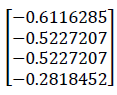The absolute values are proportional to the measures of eigenvector centrality for each vertex in the network.

Vertex                  EV Centrality
1                              .6116285
2                              .5227207
3                              .5227207
4                              .2818452

We can see, that vertex 1 has the highest measure of eigenvector centrality, followed by #2 &3, and #4 as the lowest values.

SNA with NetDraw and igraph in R

If you are not interested in actually doing the matrix algebra, or programming, you can also use the excellent point and click interface of NetDraw, developed by Steve Borgatti.  The following ‘dl’ file layout can be read into netdraw as a text file:

dl
n = 4
labels embedded
format = fullmatrix
data:
1 2 3 4
1 0 1 1 1
2 1 0 1 0
3 1 1 0 0
4 1 0 0 0

The documentation for NetDraw can be found online, and is sufficient to get started.  Reading the above textfile into NetDraw by ‘opening’ it as a ‘Ucinet DL Text File -Network (1-Mode)’ instantly creates the following visualization of the network. (Ucinet is actually another SNA software tool).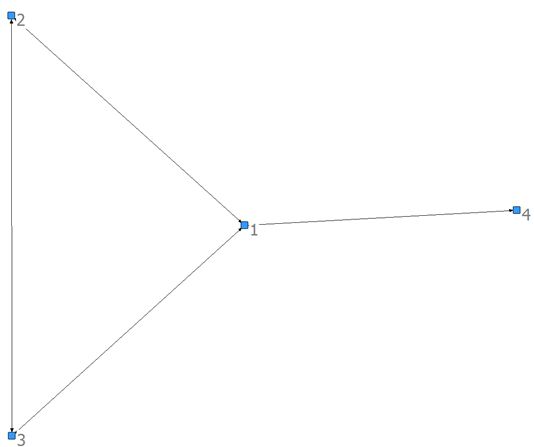Using the analysis tab in NetDraw, you can tell it to calculate various measures of centrality, including eigenvector centrality, and scale the size of the vertices based on the measure you are interested in.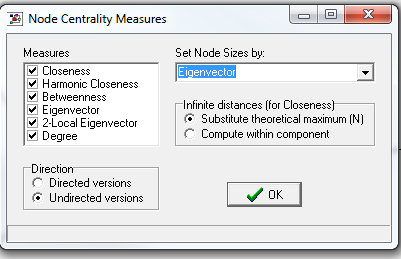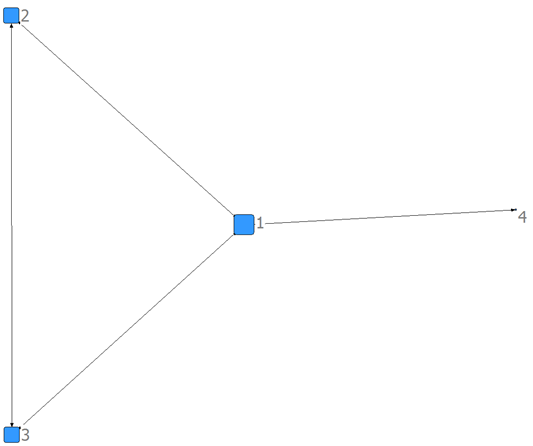There are also numerous options for changing the network vertex labels, shapes, colors etc. Using the ‘igraph’ package in R, similar visualizations can also be obtained (see the R code that follows references below).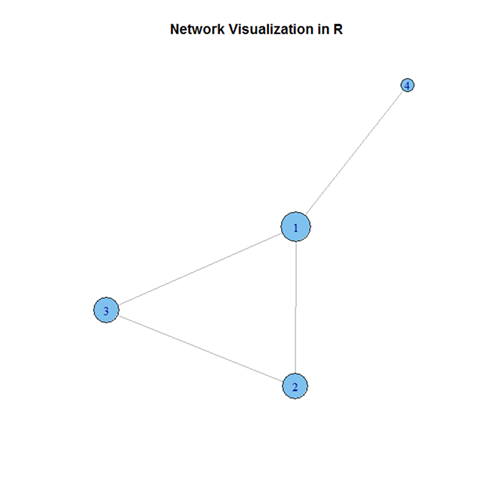In the chart below, are the comparisons of network metrics derived from matrix operations in R, Netdraw, and igraph.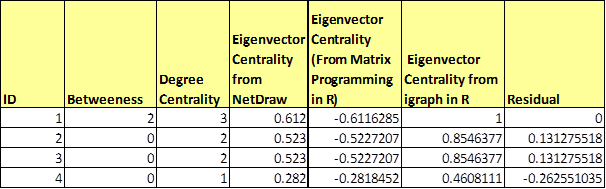Note that the absolute values of the eigenvector derived from R match the values derived from Netdraw’s computation of eigenvector centrality, while the results from igraph are simply normalized values of the eigenvector.  The residual calculation will be used in the key actor analysis discussed below.

One way to identify key actors in a network is to compare relative values of centrality such as eigenvector centrality and betweenness. Its apparent that many measures of centrality are correlated (Valente et al, 2010). If we assume a linear relationship between eigenvector centrality and betweeness and regress betweeness on eigenvector centrality, the residuals can be used to identify key players (Conway, 2009).   A vertex or individual with higher levels of betweenness and lower EV centrality may be a ‘critical gatekeeper  or an individual that is central to the functioning of the network. Someone with lower levels of betweeness and higher EV centrality may have unique access to other individuals that are key to the functioning of the network (Conway, 2009).

Based on the code provide by Conway,  the igraph and ggplot packages in R can be used to create a plot that visualizes each vertex’s relative value of EV centrality and betweeness, scaled by the value of the regression residual.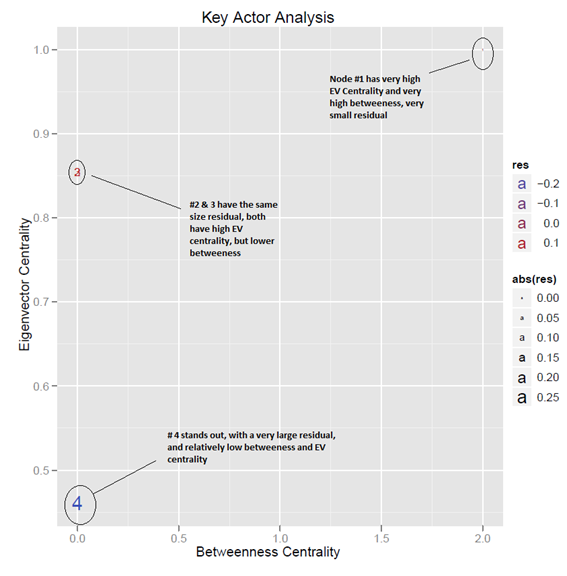Centrality measures for each individual could easily be exported and incorporated into other analytic applications such as predictive modeling. Snapshots of network metrics, as well as network structure could be examined over time to evaluate policy impacts. These basic tools are only the beginning of possibilities for applications of SNA.

References:
Matt Bogard. "Using Twitter to Demonstrate Basic Concepts from Network Analysis". Jan. 2010.
Available at: http://works.bepress.com/matt_bogard/9

Borgatti,et al. Network Analysis in the Social Sciences. Science 323. 892 (2009)

Borgatti, Steve. Basic Social Network Concepts. 2002(presentation) Boston College

Conway, Drew. Social Network Analysis in R. New York City R User Group Meetup Presentation (link) August 6, 2009

Conway, Drew. Optimal Terrorist Network Structures. (link) 2009

The mathematics of networks. M. E. J. Newman
Center for the Study of Complex Systems, University of Michigan, Ann Arbor, MI 48109–1040

International Network for Social Network Analysis .  http://www.insna.org/sna/what.html
The use of Social Network Analysis in Innovation Research: A literature review
Fabrice Coulon, PhD Candidate. Division of Innovation - LTH
Lund University, Sweden . January 17,2005. http://www.druid.dk/conferences/winter2005/papers/dw2005-305.pdf

Borgatti, S.P. 2002. NetDraw: Graph
Visualization Software. Harvard: Analytic
Technologies (Version 2.113).

How Correlated Are Network Centrality Measures?
Thomas W. Valente, PhD, Kathryn Coronges, MPH, Cynthia Lakon, PhD, and Elizabeth Costenbader, PhD Connect (Tor). Author manuscript; available in PMC 2010 May 25.
Published in final edited form as:
Connect (Tor). 2008 January 1; 28(1): 16–26.

R Code:

```# *------------------------------------------------------------------
# | PROGRAM NAME: R_BASIC_SNA
# | DATE: 4/9/12
# | CREATED BY: MATT BOGARD
# | PROJECT FILE: P:\R  Code References\SNA
# *----------------------------------------------------------------
# | PURPOSE: DEMONSTRATION OF BASIC CONCEPTS OF NETWORK ANALYSIS
# | REFERENCES: Conway, Drew. Social Network Analysis in R.
# | New York City R User Group Meetup Presentation August 6, 2009
# *------------------------------------------------------------------

#------------------------------------------------
# matrix algebra
#------------------------------------------------

A <- matrix(c(0,1,1,1,1,0,1,0,1,1,0,0,1,0,0,0 ),4,4, byrow= TRUE)
EV <- eigen(A) # compute eigenvalues and eigenvectors
max(EV\$values)  # find the maximum eigenvalue

# get the eigenvector associated with the largest eigenvalue
centrality <- data.frame(EV\$vectors[,1])
names(centrality) <- "Centrality"
print(centrality)

#------------------------------------------------
# igraph tools
#------------------------------------------------

library(igraph)

cent<-data.frame(bet=betweenness(G),eig=evcent(G)\$vector) # calculate betweeness & eigenvector centrality
res<-as.vector(lm(eig~bet,data=cent)\$residuals)           # calculate residuals
cent<-transform(cent,res=res)                             # add to centrality data set
write.csv(cent,"r_keyactorcentrality.csv")                # save in project folder

plot(G, layout = layout.fruchterman.reingold)             # network visualization

# create vertex names and scale by centrality
plot(G, layout = layout.fruchterman.reingold, vertex.size = 20*evcent(G)\$vector, vertex.label = as.factor(rownames(cent)), main = 'Network Visualization in R')

# key actor analysis - plot eigenvector centrality vs. betweeness
# and scale by residuals from regression: eig~bet

library(ggplot2)

p<-ggplot(cent,aes(x=bet,y=eig,label=rownames(cent),colour=res,
size=abs(res)))+xlab("Betweenness Centrality")+ylab("Eigenvector Centrality")
pdf('key_actor_analysis.pdf')
p+geom_text()+opts(title="Key Actor Analysis")

dev.off()```
Created by Pretty R at inside-R.org

1.2.